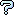All about flooble | fun stuff | Get a free chatterbox | Free JavaScript | Avatarsperplexus dot infoSquare of an Odd (Posted on 2002-10-06)Take any odd number and square it. It will invariably be a multiple of 8 plus 1. So (odd)^2=8n+1 where n is an integer. Show why this is always so. Also show what the pattern for n is.

 Submitted by martyn Rating: 3.1333 (15 votes) Solution: (Hide) Z = an even number (Z + 1) = odd n = integer (Z+1)^2 = z^2+2z+1 so we're trying to prove that z^2 + 2z + 1 = 8n + 1 so z^2 + 2z = 8n since z is even, it must be 2w where w is an integer (2w)^2 + 2(2w) = 8n 4w^2 + 4w = 8n 4*(w^2 + w) = 8n 4*[w*(w+1)] = 8n 8*[w*(w+1)/2] = 8n //notice that I turned the 4 into 8/2 [w*(w+1)]/2 = n If you multiply two integers together, and at least one of them is even, the product will be even. Therefore, w*(w+1) is even, since one of them has to be even, and one of them has to be odd. So if [w*(w+1)] is even, then [w*(w+1)]/2 is an integer, equal to the n that we started with (8n+1) It just so happens that [w*(w+1)]/2 = the sum of all the non-negative integers up to and including w (0 + 1 + 2 + 3 + ...... + w). So that is the pattern for n (0, 1, 3, 6, 10, 15). 1^2 = 8(0) + 1 3^2 = 8(1) + 1 5^2 = 8(3) + 1 7^2 = 8(6) + 1 9^2 = 8(10) + 1 11^2 = 8(15) + 1 . . .Subject Author DateAnswer Math Man 2013-06-10 16:51:54Solution Praneeth 2007-08-01 13:11:24Puzzle solution ( Continued) K Sengupta 2007-04-20 12:23:11Puzzle solution K Sengupta 2007-04-20 12:16:21sol Andre 2005-09-07 22:03:39hmm.. Amon 2005-05-04 02:46:33Similar problem Jonathan Fletcher 2004-10-07 07:32:51im smart Billy Bob 2004-03-25 13:05:15induction does it Ady TZIDON 2004-03-04 03:30:15a simple solution red_sox_fan_032003 2004-03-03 17:56:18Part 1,The formula for n Tim Axoy 2003-03-26 02:24:55Part 1,The odd number 2m+1 Tim Axoy 2003-03-26 02:19:24re: Alternate Approach Gamer 2003-03-12 14:06:00Not factorial! TomM 2002-10-07 19:16:26Square of an Odd Number Douglas Johnson 2002-10-07 15:47:22Answer to first question Dulanjana 2002-10-07 14:46:57Alternate Approach TomM 2002-10-06 17:52:23re: no solution? TomM 2002-10-06 17:33:36no solution? martyn 2002-10-06 17:19:53Heh! TomM 2002-10-06 13:25:45Please log in:

 Search: Search body:
Forums (0)